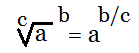# The tutor shows an example program with a few facets to discuss.

So far as I know, to find the cube root in Perl, you need use the principleThe following program calculates the cube root of a number given with the command-line function call:

\$input=\$ARGV;

if(\$input<0){
\$input*=-1; #changes value to positive
\$out=-1*\$input**(1/3);
\$input*=-1; #restores original value
}

else{
\$out=\$input**(1/3);
}
&printout;

sub printout{
print “The cube root of \$input is \$out\n\n”;
}

I’ll be explaining some ins and outs of this program next post:)

Source:

McGrath, Mike. Perl in easy steps. Southam: Computer Step, 2004.

perlmonks.org

Jack of Oracle Tutoring by Jack and Diane, Campbell River, BC.